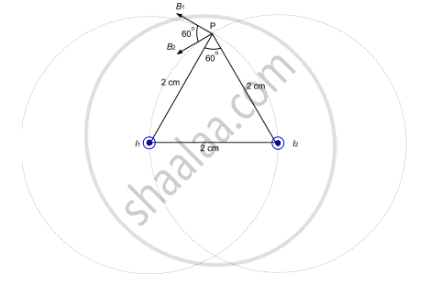Department of Pre-University Education, KarnatakaPUC Karnataka Science Class 12
Advertisement Remove all ads

# Two Parallel Wires Carry Equal Currents of 10 a Along the Same Direction and Are Separated by a Distance of 2.0 Cm. Find the Magnetic Field at a Point Which is 2.0 Cm Away from Each of These Wires. - Physics

Short Note

Two parallel wires carry equal currents of 10 A along the same direction and are separated by a distance of 2.0 cm. Find the magnetic field at a point which is 2.0 cm away from each of these wires.

Advertisement Remove all ads

#### Solution

Given:
Magnitude of currents, I1 = I2 = 10 A
Separation of the point from the wires, d = 2 cm
Thus, the magnetic field due to current in the wire is given by

$B_1 = B_2 = \frac{\mu_0 I}{2\pi d}$In the figure, dotted circle shows the magnetic field lines due to current carrying wire placed in a plane perpendicular to the plane of the paper.
From the figure, we can see that  $∆ P I_1 I_2$ is an equilateral triangle.

$\angle I_1 P I_2 = {60}^\circ$

Angle between the magnetic fields due to current in the wire, θ = 60°

∴ Required magnetic field at P

$B_{net} = \sqrt{{B_1}^2 + {B_2}^2 + 2 B_1 B_2 \cos\theta}$

$= \sqrt{\left( \frac{2 \times {10}^{- 7} \times 10}{2 \times {10}^{- 2}} \right)^2 + \left( \frac{2 \times {10}^{- 7} \times 10}{2 \times {10}^{- 2}} \right)^2 + \left( \frac{2 \times {10}^{- 7} \times 10}{2 \times {10}^{- 2}} \right) + \left( \frac{2 \times {10}^{- 7} \times 10}{2 \times {10}^{- 2}} \right)\cos60^\circ}$

$= \sqrt{( {10}^{- 4} ) + ( {10}^{- 4} )^2 + 2( {10}^{- 4} )( {10}^{- 4} ) \times \frac{1}{2}}$

$= \sqrt{3} \times {10}^{- 4} T$

$= 1 . 732 \times {10}^{- 4} T$

Is there an error in this question or solution?
Advertisement Remove all ads

#### APPEARS IN

HC Verma Class 11, Class 12 Concepts of Physics Vol. 2
Chapter 13 Magnetic Field due to a Current
Q 10 | Page 250
Advertisement Remove all ads

#### Video TutorialsVIEW ALL 

Advertisement Remove all ads
Share
Notifications

View all notifications

Forgot password?